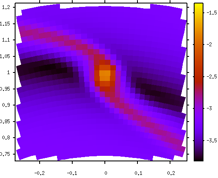# Fargnuplot## Plotting Tool for FARGO

Fargo is a simple and fast 2D polar hydrodynamic code dedicated to planet-disk tidal interactions. This perl script generates 2D plots, to quickly check the results of a run.

To plot the latest surface density file generated by fargo, you simply enter
``` ./fargnuplot in/stockholm_MJ_visc.par ```
Run ‘./fargoplot -h’ to see all available command line options and more examples. Once the plot is created, you end up in gnuplot, where you can make further processing such as zooming (use right mouse button in X11 window), saving the plot (“set terminal postscript; set output ‘out.ps’; replot”) or changing the colour map. You can even run this script while fargo is running to analyze the latest files. You don’t have to compile anything. But note that this script needs a gnuplot version 4.2 or newer that supports binary files.

## Usage

``` Usage: fargnuplot [options] [in/fargo.par | directory]```

```-h print this message -d do not delete temporary files, usefull for further processing -p persistent X11 window -x exit gnuplot after plotting (implies -p) -num=NUM plot file with number NUM in given directory instead of newest file -file=FILE plot FILE, need to specify nr and ns as well -nr number of radial grid cells -ns number of azimuthal grid cells -nr1D number of radial grid cells in the 1D grid```

``` -g plot gas density (default) gasdens*.dat -vr plot radial velocity gasvrad*.dat -vt plot azimuthal velocity gasvtheta*.dat -t plot gas temperature gasTemperature*.dat -l use logarithmic scale -c circular plot (default: rectangular plot) -clean plots only the data, no borders, no axis -curve plot average values as a function of radius -all plot also the 1D grid (for FARGO-2D1D) -s square plot -png output png file and exit gnuplot -eps output eps file and exit gnuplot ```

```Examples: fargnuplot -nr=384 -ns=384 out5 => plot the lates density file in the directory out5 fargnuplot in/stockholm_MJ_visc.par => plot the latest density file in the output directoty specified in the .par file fargnuplot -nr=128 -ns=384 -file=out5/gasdens125.dat => plot the specified file ```

## Changelog

fargnuplot-0.42 (All thanks to Tobias Müller)
– Added -temp=DIR for specifying a directory for temporary files
(ideally a tmpfs filesystem for speed up)
- Added -q parameter for plotting of gasToomre files
- Added -lcb parameter for logarithmic scale of cb axis
- Added -r=RANGE for specifying the cb range, e.g. 0:1
- Added a handler for SIGINT (Ctrl+C)
- Added check of gnuplot’s exit code
- Added code for cleaning up temporary files
- Added lots of coments in source code
fargnuplot-0.41
- Fargnuplot can now plot the square plots (-s option).
- Fargnuplot can now output png and eps files.
- Fargnuplot uses a random hash for the temporary files to avoid conflicts.
Thanks to Tobias Mueller for all this.
fargnuplot-0.40
- There was a problem with large grid sizes due to a rounding error issues
which has been resolved.
- Fargnuplot can now plot the 1D grid as well (use options -all -nr1D=NUMBEROF1DGRIDCELLS).
Thanks to Aurelien Crida for this.
- The -num command line option works also with -num=0.
Thanks to Tobias Mueller for this.
fargnuplot-0.31
- Now works with FARGO2D1D (1D cells are ignored for now)
fargnuplot-0.30
- Added ‘-curve’ feature to plot average values as a function of radius.
- fargnuplot is now processing the used_rad.dat file if possible to determine the radiual spacing.
fargnuplot-0.20
- Added logarithmic scaling and circular plot.
fargnuplot-0.10
- Inital release.